0

## GHOST Busting

Published on Sunday, July 28, 2013 in , , , ,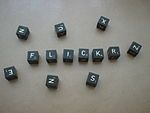As you may know from my Nim posts, I'm fond of games that can be analyzed to assure an advantage over someone.

Instead of yet another version of Nim, however, today we're going to look at another classic game, known as GHOST. It's a verbal word game, so the only item needed is some sort of resource, such as a dictionary, which will dictate which words are legal, and which words are not.

There are several variations of GHOST, but I'll only be focusing on the classic version of the game. In order to assure an advantage, I'll also only be focusing on a game with 2 players in American English.

Here are the rules of GHOST that apply to our 2-player game, as explained on Wikipedia's GHOST (game) page:

A player is chosen at random to start the game, and begins by naming any letter of the alphabet. Players then take turns to add letters to this fragment, with the aim being to avoid completing an actual word. The player whose turn it is may - instead of adding a letter - challenge the previous player to prove that the current fragment is actually the beginning of a word. If the challenged player can name such a word, the challenger loses the round; otherwise the challenged player loses the round. If a player bluffs, or completes a word without other players noticing, then play continues. When a round ends, play generally passes to the left.

If any score is kept at all, the traditional method uses the letters of the word "Ghost" in the same fashion as the basketball game horse, with each loss giving the player the next letter of the word, and a player being eliminated when they have all five letters.

It's easy to see how Nim can be analyzed mathematically, but how is a game like this possible to analyze when we're restricted to words of a given language?

In a 2-player game, the first player can win by forcing the 2nd player to complete a word with an even number of letters. Similarly, the second player can win by forcing the 1st player to complete a word with an odd number of letters.

As I mentioned above, there must be some sort of reference book used as the standard for legitimate words. Traditionally, when playing this game, you'd carry around a dictionary. Today, it's easier to play with a reference which is accessible from a mobile device. Over in zyzzyva.net's word list page, there are several good word lists, with definitions, that will work for this. For reasons that will be explained later, I highly recommend the Official Scrabble Player's Dictionary 4 list.

Once you have a standardized list, it's possible to work out your best strategies. How? Basically, you're looking for letter combinations that limit the choices to only odd (for strategies where you're the 2nd player) or only even choices (for strategies where you're the 1st player).

This basic idea was first discussed in Word Ways in the early 1970s in a piece titled “Our Move”. It was written about again, in “Pocket Ghost”, but the most impressive work done was by Alan Frank in 1987, using the Official Scrabble Player's Dictionary (OSPD), in “Ghostbusted”.

Alan Frank's usage of the OSPD is why I recommended that particular word list link above. Do note that Mr. Frank considered words from there that only used up to 9 letters, while the linked word list included words up to 15 letters. You'll probably want to either verify that the strategies still work with the OSPD4 list and/or whittle down the list to insure your advantage. If you do the latter, you'll obviously need to store it somewhere on the web, and give it an easy-to-type and easy-to-remember link.

Once you have a set of words with which you're comfortable, how do you access them? Not surprisingly, I advocate memorizing the needed words. This sounds like plenty of work, but if you use the Link System, as taught in these videos, and memorize the words in order from shorter to longer, then the process can be more fun than you probably thought.

Challenge yourself to win, and then challenge your friends. If you've found any resources that aid in winning at GHOST, I'd love to hear about it in the comments!1

## Making Math Tricks Easier

Published on Thursday, July 25, 2013 in , ,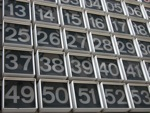In many mental math shortcuts, and much of math, the processes work better with some numbers than with others.

For example, it's easier to work out the square root of 49 in your head than it is to work out the square root of 58. When dividing by 9, 72 is easier to work with than, say, 83.

In this post, you'll learn a simple yet powerful technique that can tame the more challenging numbers. Once you learn this and apply it, you'll find it can make many other math tricks easier!

BASICS: The basic idea is simply to break up the more challenging numbers into an addition problem, and deal with each part in a different way. You don't just choose any two numbers, however. The real trick is in creating the addition problem wisely.

The very first step is consider what kind of numbers work easier in the type of problem with which you're dealing. If you're going to be dealing with square numbers, then obviously knowing the perfect squares will be of great help. Perhaps you're dealing with divisibility by 7, in which case the multiples of 7 would be important.

When you have a given number, and you understand the type of numbers you need, find the largest number that is easy to deal with which is less than or equal to the given number, and use that as the first number in the addition. We'll call this first number the “reference number.” The other number is simply the distance from the reference number to the given number, so we'll call that number the “distance.”

To clear things up with a practical example, imagine you're challenged to give the square root of 51. Since we're dealing with square roots, we're looking for a perfect sqaure. What's the largest perfect equal to or less than 51? It's 49, so 49 becomes our reference number. We were given the number 51, which is 2 numbers (the distance) after 49, so we would break 51 down into the addition problem of 49 + 2.

From here, you would deal with 49 in one way, and 2 in another way. If you're actually interested in learning the square root process, I explained it in this blog past last year.

As another example, let's say divisibility by 9 is important in a particular process. In this case, we know we need to focus on multiples of 9. Let's take the same example number from above, 51, and break it down by focusing on divisibility by 9. The largest multiple of 9 less than or equal to 51 is 45, which is 6 away from 51. This means that, for this particular task, you'd break 51 up into 45 (the reference number) + 6 (the distance). As before, you'd deal with the 45 with one approach, and the 6 in another, depending on the process. When multiplying by 10/9, as taught in my recent post, A Trick For Temperatures, you'll find that this approach comes in very handy.

Notice that the same number, 51, was broken up in different ways, depending on what features were important. As long as you know what features of a number are important, the actual process of breaking up the number is relatively simple.

MODULUS: Modular arithmetic is used in many math tricks, and this approach makes it ridiculously easy. If you need to refresh yourself on the basics of modular arithmetic, read this betterexplained.com post.

Let's say the problem we have to deal with is 51 mod 4. The mod 4 portion of the problem tells us right away that multiples of 4 are important. What's the largest multiple of 4 equal to or less than 51? 48 of course! So, 48 becomes our reference number, and 3 is the distance. We've broken this problem down into (48 + 3) mod 4. What's next?

For modulo calculations, the process is ridiculously simple: Ignore the reference number completely, and use the distance as your answer!

In our example of (48 + 3) mod 4, that means we completely ignore the 48, and that our answer is 3! We went from 51 mod 4 to (48 + 3) mod 4 to simply 3.

How about 51 mod 9? You should already understand why 45 would be the reference number, and 6 is the distance, so this problem becomes (45 + 6) mod 9. Ignoring the reference number, 45, we use the distance, 6, as our answer.

Modulo 9 is very useful in mathematics. In fact, it has a strong connection with what are known as digital roots (YouTube video). Calculating any number mod 9 will give you an answer ranging from 0 to 8, yet digital roots are always in the range of 1 to 9. When you take a number and perform mod 9 on it, and get an answer from 1 to 8, that's also the digital root, but if you get 0 as an answer, the digital root is 9.

For example let's try 72 mod 9. 72 is already a multiple of 9, so the reference number would be 72, and the distance would be 0, turning the problem into (72 + 0) mod 9. Obviously, the answer here is 0. However, the digital root would be 9, as explained in the previous paragraph.

NUMBER BASES: This trick becomes essential when working with numbers in different bases. Just last month, I featured an entire post on hexadecimal numbers, which is base 16.

When dealing with 2-digit hexadecimal numbers, it's important to know your multiples of 16 from 0 up to 15 by heart. Combined with your knowledge of this reference number and distance trick, 2-digit hexadecimal number become a lot easier to tame.

What is 189 in hexadecimal? Let's break it down into a sum first. What's the largest multiple of 16 which is less than or equal to 189? 176, so that's our reference number. The distance from 176 to 189 is 13, so 189 becomes 176 + 13.

With hexadecimal, you divide the reference number by 16, and leave the distance as it is. Both of these number are then translated into letters (as taught here), if they represent numbers from 10 to 15. The two number are put side by side, and you have your hexadecimal equivalent!

So, we've broken 189 up into 176 + 13. We take the reference number 176, and divide that by 16 to get 11. Since multiples of 16 are so important, this could actually be done from memory. We have 11 and 13 at this point. 11, in hexadecimal, becomes a B and 13 becomes a D, so now we have BD. That means the decimal number 189 is the same as the hexadecimal number BD!

How about 152? It should break up into 144 + 8. Do you understand why? 144 ÷ 16, as we should recall from knowing our multiples of 16, is 9, so we have 9 and 8. No conversion into letter is required, so decimal 152 equals hexadecimal 98.

For working with any base n, you should known all the multiples of n from 0 to n-1. When you get the reference number, knowing all these multiples by memory will help make you division of the reference number by n much faster. For example, when dealing with base 8, you should know all the multiples of 8 from 0 to 7 by heart, so dividing the reference number by 8 becomes a matter of memory, and therefore quicker to perform mentally.

FINAL THOUGHTS: There are some rare cases where you may need to break up the given number into a subtraction problem, instead of an addition problem. In the year section of my moon phase tutorial, you need to find how close a year is to the nearest multiple of 19, whether that multiple is larger or smaller than the given year.

Using the example of 13 from the tutorial, you might be tempted by the instruction above to break 13 up into 0 (the largest multiple of 19 less than or equal to 13) + 13 (the distance from 0). However, in the case of the moon phase tutorial, you should break it up into 19 - 6, with 19 being the reference number (13 is closer to 19 than it is to 0), and - 6 being the distance.

Again, the key is properly understand any math trick before applying this approach.

With a little thought and creativity, you should be able to adapt this reference number and distance technique to your favorite math tricks, and make them easier in the process. I would love to hear about any uses you have for this approach in the comments below!0

## Yet Still More Quick Snippets

Published on Sunday, July 21, 2013 in , , , , , ,July's snippets are ready!

Instead of trying to pick a theme this month, I decided to return to the original purpose of snippets, and just grab random items of interest to Grey Matters readers:

• For those who enjoyed my post on temperature conversion, and the added trick to make it easier, there are simple tricks for other metric conversions, as well.

Nurse Debra Mallory teaches this simple trick for converting pounds to kilograms. Need to work out a metric distance? Here's a quick trick for converting kilometers to miles.

Last month's snippets focused on hexadecimal numbers. If you want to learn more about those, check out this introduction to hexadecimal numbers. The approach taught on that page is taught quite clearly, so it's easy to grasp.

• Many of the feats I teach on this site require mental arithmetic. If you want to take your mental math to the next level, check out Aaron Maxwell's online book Inner Algebra: How To Do Algebra In Your Head. The whole book is available at that link, and you can also buy a paperback copy on Amazon.

• Just for fun, and completely unrelated to just about anything else I've ever posted on this site, check out this fun and amazing toy, known as Tumble Rings. You can build them yourself, using several sets of key rings. If you're familiar with the toy known as a Jacob's Ladder, you'll recognize the same principle at work here:

That's it for July's snippets. If you have anything you'd like to add, let me hear about it in the comments below!0

## Divide and Conquer

Published on Thursday, July 18, 2013 in , , , , , ,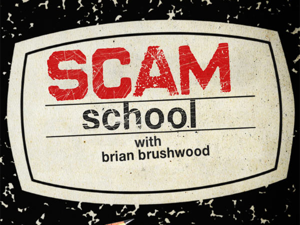Ever try and solve a puzzle involving physical objects, such as cards, coins, or chips, with your eyes closed?

Despite the challenging conditions, this is just what you're going to learn in today's post! Even better, the solution is ingeniously simple.

Let's get right to the puzzle, shall we? It's presented in its basic puzzle form in this week's episode of Scam School. Before you play the video, grab 10 cards, and try and work out the puzzle before viewing the solution.

Did you solve it on your own? If so, congratulations! Even if you didn't, you should at least have viewed the solution before moving on from this point.

Now, let's try a similar puzzle, but we're going to throw a bit of a curve into it. Add another card to your group of 10, so you now have 11 cards. Just as before, mix them so that some are face-up and some are face-down. You challenge is to separate these 11 cards into 2 groups, so that both groups contain the same number of face-up cards. James Grime has a video puzzle version of this, using chips instead of cards.

Why is this version so much more challenging? There's no way to divide 11 into 2 piles of the same number of cards (or chips, coins, etc.), and that was such an important feature of the previous version.

Once again, try working it out for yourself with cards in hand, before peeking at the solution. When you're ready, here's James Grime with the solution to dealing with odd numbers of objects:

As you see, you need extra information to solve this latter version of the puzzle.

While this does make an interesting puzzle, it can also be turned into a magic trick quite easily. Bob Hummer and Jack Yates created a trick using this principle called Time Will Tell. That version uses 12 pennies, arranged in a clock formation, with some marker identifying one of the pennies as the 12 o'clock position. The performer turns away from the proceedings, and asks the volunteer to turn over any 6 pennies. Without looking at which coins were flipped and without asking any questions, the performer then separates the pennies into 2 groups, both with the same number of heads and tails in each group.

In the original version of the trick, you set up all 12 coins with heads up. As you've seen, however, this really doesn't matter. They can start with any number of heads and tails showing, and have them do several prescribed flips, and you can still separate the groups into 2 sets with equal amounts of heads and tails. Instead of simply having your back to the proceedings, you can even do this over the phone.

Whether you see it as a puzzle or a magic trick, this is a fun exercise to analyze and figure out why it works. If you develop any interesting versions of this routine, I'd love to hear about it in the comments!0

## Secrets of Nim (Visual Multi-Pile Nim)

Published on Sunday, July 14, 2013 in , , ,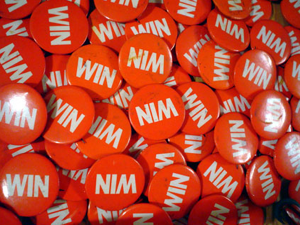It's been a while since we've visited our old friend Nim.

Instead of a new version, we're going to a new look at the classic multi-pile Misère Nim.

If you need a refreshed course, I taught the basics of standard multi-pile Nim here, and the basics of multi-pile Misère Nim here.

The math in those articles did get somewhat technical, so I later taught a better way to visualize the board in order to help you see your best strategy.

Earlier this year, YouTube user Hythloday71 posted the video directly below, which discusses multi-pile Misère Nim. In the video, he plays the game at this site, which requires the Flash plug-in. If you're on a device without the Flash plug-in, you can play the match version here. Try an see if you can figure out how to win, before moving on.

What really drew me to this video in particular, however, was the explanation video. In my earlier visualization post, I tried illustrating the idea in text. In the video below, however, the same visualization concept is presented with straw frames of different colors that really bring the concept to life!

If you enjoyed this game, check out the other Nim posts on Grey Matters. Since Nim is a game you can always win, and has so many variations, it's fun to explore!0

## Prime Time

Published on Thursday, July 11, 2013 in , ,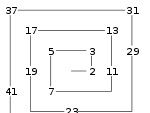Today's date, 7/11/13 is interesting, as it consists solely of consecutive prime numbers. The only other times this century that this will happens are on 2/3/05, 3/5/07, 5/7/11, and 11/13/17.

Since the date brings us a prime pattern, today seems like a good day to look at prime patterns.

With help from Brady at numberphile, James Grime starts us off by explaining the concept of prime spirals:

Numberphile didn't stop there, though. Starting from a passage in one of Arthur C. Clarke's book, the following video discusses the importance of the number 41 to Ulam's spiral:

While they don't show every step in the video, Wolfram|Alpha can show you all the results of n2-n+41 quickly and easily.

I hope pondering these interesting patterns gives you plenty of food for thought!2

## Doomsday Improvements

Published on Sunday, July 07, 2013 in , , , ,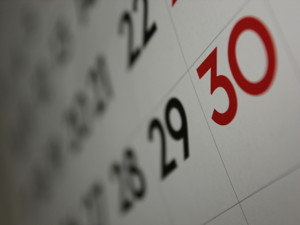Doomsday Improvements - I bet those are two words you never expected to see together!

Doomsday, in this context, refers to the Doomsday algorithm developed by John Conway, which helps determine the day of the week for any given date. Since it was originally published in 1973, there have been many variations and improvements, and we'll look at some clever ones in this post.

The classic Doomsday Algorithm starts by determining the day of the week in a given year on which the last day of February falls. From there, you're able to work out any date in the rest of the same year.

There are other approaches, of course. Another popular approach for calendar calculation returns results from 0 to 6, where 0 represents a Sunday, 1 represents a Monday, and so on all the way up to 6, which represents a Saturday. Effectively, these methods are based on working out how far a date in a given month and year are from the first Sunday. We can call this a “First Sunday” approach, to differentiate it from the Doomsday approach.

The great thing about the Doomsday approach, of course, is how easy it makes some of the calculations. The great thing about the First Sunday approach is the ease of interpreting the results. Back in 2009, Bob Goddard combined the best of both worlds with his First Sunday Doomsday (FSD) algorithm!

If you've learned the Doomsday approach, you can quickly adapt to this new approach. Let's take a close look at it, and maybe even improve on it.

YEAR CALCULATION: Step 1 determines a key for the year, based on the popular Odd + 11 approach, described on Grey Matters here, and on Wikipedia here. If you read carefully, however, there is an important difference.

The last step of the standard Odd + 11 approach requires you to subtract the result of the previous steps from 7. In the FSD doomsday approach, this step is skipped entirely! Naturally, the fewer the calculations, the quicker you can get to the result.

In 2008, Mike Walters published a quicker way to get the same result as Odd + 11 that would work well here. You can read it in detail at that link, but I'll describe the practical steps here.

Note that Mike Walters' approach also involved subtracting from 7 as the last step. Since it's not used in Bob Goddard's version of Odd + 11, we also won't be using that subtraction from 7 here, either. That way, the results remain consistent.

When given a year, ask yourself whether the last two digits are evenly divisibly by 4. If they aren't, keep adding 11 years at a time, until you get to a year that is evenly divisible by 4. You'll only ever have to add 11, 22, or 33 years, and you don't need to worry if the total goes over 100. Once you're dealing with a year which is evenly divisible by 4, divide that year by 2, and then subtract the nearest multiple of 7 which is equal to or greater than that number. You're done!

Let's use Mike Walters' example of 1953, so we'll only focus on the 53. 53 itself isn't evenly divisible by 4, so we add 11 and get 64, which works! 64 divided by 2 is 32, and 32 - 28 (the nearest multiple of 7 equal to or less than 32) = 4, we now know our key number for the year is 4!

Notice that in Mike Walters' write-up, he gets 3 instead of 4 for 1953? Again, that's because Bob Goddard's FSD approach differs from the standard Doomsday approach by removing the final subtraction from 7. Removing this final step from Mike Walters' approach ensures that we get the correct results required for the FSD approach.

In practice, any even number which is NOT evenly divisible by 4, such as 1962, just needs to have 22 added (62 + 22 = 84). Also, any odd numbered years, such as 1933 or 1947, will require either 11 or 33 to be added to get to a multiple of 4 (33 + 11 = 44, and 47 + 33 = 80).

CENTURY CALCULATION: This is where Bob Goddard's FSD approach really shines! As you may know, the Gregorian calendar repeats exactly every 400 years. Bob Goddard's approach uses the 1700s as a basic century, and the century keys adapt from there, using his ingenious “No tune for Friday” mnemonic.

The 1700s are used as the basic century for 2 reasons. First, because the Gregorian calendar wasn't used in Britain and its colonies (including America) until 1752. Second, it's easy to think of the 1700s as the century in which America got its start, so you can think of the Gregorian cycle as an American history cycle, too.

The astounding thing about the FSD algorithm, though, is that it handles Julian dates easily! Instead of using the 4 century pattern for Gregorian dates, you simply use the century number for Julian dates. For the 1400s, you add 14, and for the 900s, you add 9. It's surprisingly simple.

If you're familiar with how the traditional Doomsday approach handles months, you'll note the same mnemonics are used in the FSD approach, so that's an easy adaption.

If previous approaches have been giving you trouble, try Bob Goddard's FSD algorithm. You just may be surprised how quickly you can pick it up.0

## A Trick For Temperatures

Published on Thursday, July 04, 2013 in , , ,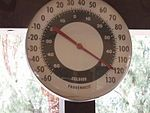Last month, I discussed how to convert between Celsius and Fahrenheit, including how to remember the correct steps.

There was one major fly in the ointment. When going from °F to °C, you have to multiply by 109, or 1.111..., which seems difficult to do in your head. In today's post, however, you'll learn how to perform this calculation easily!

MULTIPLES OF 9: When you think about it, multiplying by 109 is really just multiplying by 10 and then dividing by 9. When dealing with multiples of 9, this is very easy. What is 109 of 9? 109 × 9 = 909 - 10. Working through all the 1- and 2-digit multiples of 9, we get:

• 109 × 9 = 10
• 109 × 18 = 20
• 109 × 27 = 30
• 109 × 36 = 40
• 109 × 45 = 50
• 109 × 54 = 60
• 109 × 63 = 70
• 109 × 72 = 80
• 109 × 81 = 90
• 109 × 90 = 100
• 109 × 99 = 110
When dealing with tempertures of 158 °F or less, which is well over the hottest air temperature ever recorded on Earth, you're only going to be dealing with 2-digit numbers, so I'll be limiting this tutorial to 2-digit numbers.

If you're lucky enough to be working with a multiple of 9, you already have your answer at this point!

Besides being easy to remember, the multiples of 9 are also helpful in another way. If you're calculating with a number from, say, 50 to 59, is the answer going to be in the 50s or the 60s? Anything in the 50s below 54 would have to give an answer in the 50s, and any number in the 50s equal to or greater than 54 would have to give an answer in the 60s.

Using this technique, you can already say the first half of the answer without calculation! What is 109 of 73? Since we already know 72 would take us to 80, then so must 73, so we can start by saying, "Eighty...". How about 33? 36 takes you to 40, so 109 × 33 must still be in the 30s somewhere. If you say, "Thirty..." at this point, you already have the first half of your answer!

Now that we've narrowed down the answer to a specific range of 10 numbers, how do we get the rest of the answer?

DIGITAL ROOTS: The original number itself will clue you in to the rest of the answer in a surprisingly easy manner. To get the second half of the answer, you need to use what is known as the digital root.

The digital root of a number is always a 1-digit number from 1 to 9, and is calculated by adding the digits of the number together. For example, the digital root of 43 is 7 because 4 + 3 (the digits in the original number) = 7. Sometimes, you may have to repeat this process to get a 1-digit number. To find the digital root of 75, you would add 7 + 5 = 12, and then repeat the process with the 12, 1 + 2 = 3. So, the digital root of 75 would be 3.

Another way to get the digital root of a number is to subtract the nearest multiple of 9 that is less than or equal to your number. With 43, the nearest multiple of 9 less than or equal to it is 36, and 43 - 36 = 7. Similarly, with 75, 75 - 72 = 3.

You can learn about digital roots in more detail in this thonky.com post, as well as by watching this video.

Working out the digital root will tell you the final digits of the answer, in this way:
• A digital root of 1 will end in 1.111..., or 119
• A digital root of 2 will end in 2.222..., or 229
• A digital root of 3 will end in 3.333..., or 339, or 313
• A digital root of 4 will end in 4.444..., or 449
• A digital root of 5 will end in 5.555..., or 559
• A digital root of 6 will end in 6.666..., or 669, or 623
• A digital root of 7 will end in 7.777..., or 779
• A digital root of 8 will end in 8.888..., or 889
• A digital root of 9 will end in 0 (as discussed above)
This is a very easy pattern to remember, of course. This also gives you the precise second half of your number!

Let's work through the whole process now with some examples. What is 109 × 43? 43 is less than 45, so we know our answer will stay in the 40s, so we can already say, "Forty...". The digital root of 43, as explained above, is 7, so now we know that it will end in 7.777..., and we can finish by saying, "...seven point seven seven." If we make the same calculation with Wolfram|Alpha, you see that this is correct!

What about 75? 75 is greater than 72, so we already know our answer will be in the 80s, and say, "Eighty...". The digital root of 75 is 3, so we can finish by saying, "...three point three three." Sure enough, 109 × 75 is 83.333...!

Once you become comfortable with the process, it almost seems like someone else is making the calculation, and you're just listening to the answer as it comes out of your own mouth! What is 109 × 92? It's more than 90, so you can already say, "One hundred and...", and the digital root is 2 (9 + 2 = 11, 1 + 1 = 2), so you say, "...two point two two." Being able to give such decimal precision so quickly makes this seem impressive, as well.

NUMBERS ENDING IN .5: Since this calculation is used for temperatures, and dividing by 2 is so important when going from °F to °C, you're occasionally going to deal with numbers ending in .5, such as 62.5. You handle these in almost exactly the same way, but with one important change.

Just as before, start by asking yourself where the number is in relation to the multiple of 9. With 62.5, it's below 63, so the answer will be in the 60s. You say, "Sixty..." and then work out the digital root of 62.5: 6 + 2 + 5 = 13, and 1 + 3 = 4, so the number has a digital root of 4. However, instead of using the same numbers as we did previously, a number ending in .5 requires that you use these different final digits:
• A digital root of 1 will end in 6.111..., or 619
• A digital root of 2 will end in 7.222..., or 729
• A digital root of 3 will end in 8.333..., or 839, or 813
• A digital root of 4 will end in 9.444..., or 949
• A digital root of 5 will end in 0.555..., or 059
• A digital root of 6 will end in 1.666..., or 169, or 123
• A digital root of 7 will end in 2.777..., or 279
• A digital root of 8 will end in 3.888..., or 389
• A digital root of 9 will end in 5
Note that the digits after the decimal point are still the same as the digital root, but the number immediately preceding the decimal point always has a difference of 5 from the digital root. A calculation involving .5 should remind you that you need to add or subtract 5 (whichever is easier) from the digital root for the number in the ones place (the number immediately preceding the decimal point).

Finishing up with our 62.5 example, we've already said, "Sixty..." and know the digital root is 4, so we finish by saying, "...nine point four four." Yes, 109 × 62.5 is 69.44.

How about 87.5? It's above 81, so the answer will be in the 90s, it involves a .5, and the digital root is 2 (8 + 7 + 5 = 20, 2 + 0 = 2), so we give our answer as, "Ninety...seven point two two." Do you understand how we worked through that? If not, go back and read through the process before moving on. Also, feel free to ask questions in the comments below.

TEMPERATURE CALCULATIONS: Let's use our newfound ability to precisely calculate 109 of any 2-digit number by working through the full original process from °F to °C.

What is the Celsius equivalent of 82°F? First, we add 40, so 82 + 40 = 122. Next, we divide by 2, so 122 ÷ 2 = 61. 109 of 61 is, "Sixty...seven point seven seven" (67.777..., do you understand how we got that number?). The final step is to subtract 40, so 67.777... - 40 = 27.777..., so 82°F should be about 27.77°C, and Wolfram|Alpha verifies that this is correct!

How about 75°F? 75 + 40 = 115, and 115 ÷ 2 = 57.5. Make sure that you remember the rules for .5 here! 57.5 is more than 54, and has a digital root of 8 (5 + 7 + 5 = 17, 1 + 7 = 8), so 109 of 57.5 is, "Sixty...three point eight eight." Subtracting 40 in the last step, we get 63.88 - 40 = 23.88. Sure enough, 75°F is 23.88°C!

TIPS: Here are a few ideas that can help improve this process.

If you're dealing with a 1-digit number, it's digital root is itself. The digital root of 1 is 1, the digital root of 2 is 2, and so on. With 2-digit numbers, if one of the digits is either a 0 or a 9, you can ignore those, and use the remaining digit. Ignoring the 0 in 20 gives you 2, which is the digital root of 20. Ignoring the 9 in 49 gives you a digital root of 4, and ignoring the 9 in 96 gives you a digital root of 6.

You can also ignore pairs of numbers that sum up to 9, which is especially handy when dealing with numbers ending in .5. What's the digital root of 63.5? Well, since 6 and 3 equal 9, we can ignore those, and see that the remaining 5 gives us a digital root of 5!

Again, if you have any questions about clarifying this process, feel free to ask them in the comments!